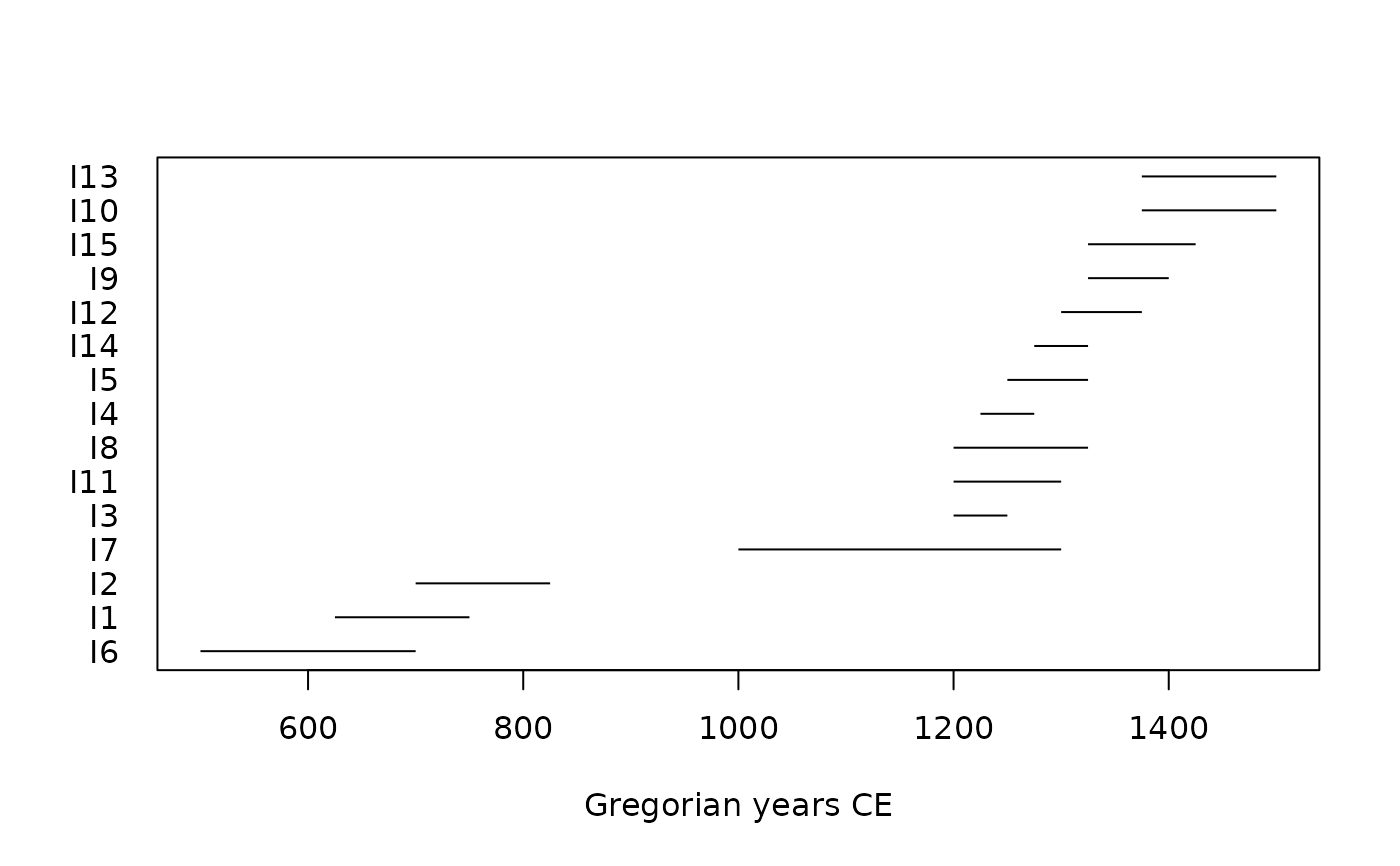Checks if intervals overlap.

## Usage

overlap(x, y, ...)

# S4 method for numeric,numeric
overlap(x, y)

# S4 method for ANY,missing
overlap(x)

## Arguments

x, y

A numeric vector giving the lower and upper boundaries of the time intervals, respectively. If y is missing, an attempt is made to interpret x in a suitable way (see grDevices::xy.coords()).

...

Currently not used.

N. Frerebeau

## Examples

## Seven intervals
i <- data.frame(
start = c(1, 2, 3, 6, 9, 13, 17),
stop = c(7, 4, 15, 14, 11, 18, 19),
row.names = c("A", "B", "C", "D", "E", "F", "G")
)

## Do the invervals overlap?
j <- overlap(i)

## Interval graph
graph <- as_graph(j)
plot(graph)